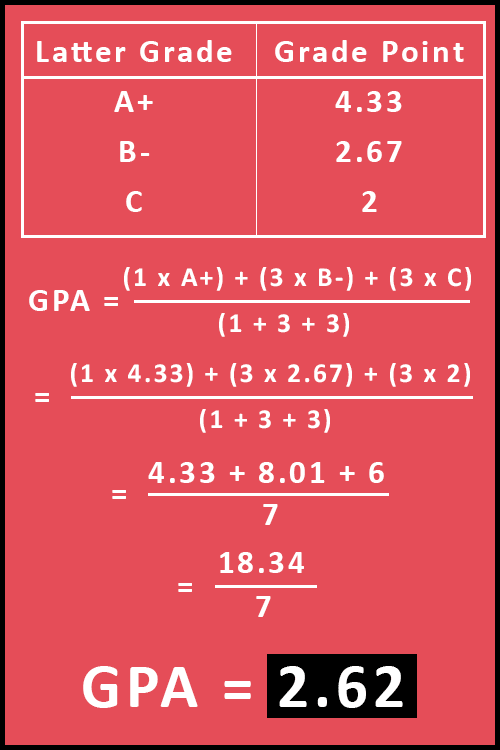# How to Calculate GPA?

If you want to know how to calculate GPA, then this article is for you. Here we will discuss some mathematics formulas and GPA calculation with some examples.

The GPA is equal to the sum-up of all the credit hours (C) multiply by grade points (G) and then divide by the sum of credit hours.

GPA =
(C1 × G1) + (C2 × G2) + ... + (Cn × Gn)
(C1 + C2 + ... + Cn)

Where,
C = Credit Hours (The hours assigned to a particular course)
G = Grade Points (You can find it from the GPA Scale Table)

Basically, there are three operations that we need to perform respectively.

• Multiplication
• Division

Now follow the step-by-step instructions to calculate the GPA.

1. Firstly, find the grade points from GPA Scale Table.
2. Multiply each credit hour and grade point and then sum up all the resultant values.
3. After that, find the sum of all the credit hours.
4. Lastly, divide step 3's value with step 2's value.

Finally, you will get the final Grade Point Average.

Now let's take some examples to understand the above all points step by step.

### Example 1:

Suppose John completed 3 courses: Maths with "A" grade, Chemistry with "B+" grade, and Physics with "B" grade. Find out the GPA.

Maths A 3 3 × 4 = 12
Chemistry B+ 4 4 × 3.33 = 13.32
Physics B 2 2 × 3 = 6
Total - 9 31.32
GPA (31.32 / 9) = 3.48

Now let's figure out how it is calculated.

Firstly, we will use GPA Scale Table to convert letter grades into grade points and then apply them to the formula.

You can see the full calculation below:### Example 2: